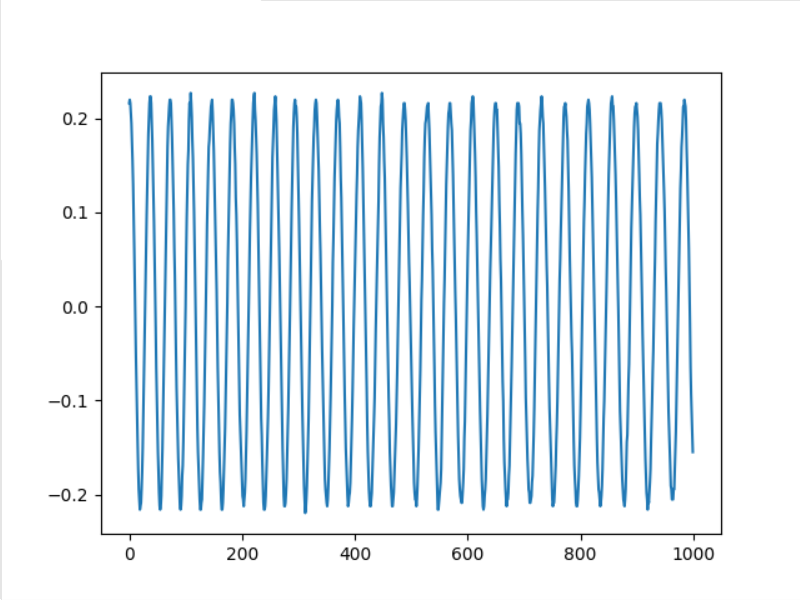• python读取.dat文件
千次阅读
2021-08-23 10:22:59

问题：用UE编辑器打开文件后，发现分隔符是“ETX”

目的：将.dat文件读取至python，机构化数据

实现：

（2）np.fromfile(r"D:\data\test.dat",dtype=... )

更多相关内容
• ## python读取dat文件

千次阅读 2021-12-31 21:22:17
import  pandas  as pd
#1：读取指定行
print("----读取指定的单行，数据会存在列表里面----")
data=df.loc.values#0表示第一行 这里读取数据并不包含表头，要注意哦！
print("读取指定行的数据：\n{0}".format(data))

print("\n------读取指定的多行，数据会存在嵌套的列表里面----------")
data=df.loc[[1,2]].values#读取指定多行的话，就要在loc[]里面嵌套列表指定行数
print("读取指定行的数据：\n{0}".format(data))

print("\n----------------读取指定的行列-----------------------")
data=df.iloc[1,2]#读取第一行第二列的值，这里不需要嵌套列表
print("读取指定行的数据：\n{0}".format(data))

print("\n----------------读取指定的多行多列值-----------------------")
data=df.loc[[1,2],['title','data']].values#读取第一行第二行的title以及data列的值，这里需要嵌套列表
print("读取指定行的数据：\n{0}".format(data))

print("\n-----------获取所有行的指定列----------------------------")
data=df.loc[:,['title','data']].values#读所有行的title以及data列的值，这里需要嵌套列表
print("读取指定行的数据：\n{0}".format(data))

print("\n------------获取行号并打印输出---------------------------")
print("输出行号列表",df.index.values)

print("\n-------------获取列名并打印输出--------------------------")
print("输出列标题",df.columns.values)

print("\n------------获取指定行数的值---------------------------")

print("\n-----------获取指定列的值----------------------------")
print("输出值\n",df['data'].values)


#获取总行数
lines=df.shape
#查看各列数据类型
print(df.dtypes)


#读取dat文件
#delimiter：读取具有分隔符的数据
#dtype：第0，5，19列以字符串格式读取

#写入dat文件
#index=0:索引不写入
#sep='|'：写入具有分隔符的数据

展开全文开发语言 后端
• 摘要：主要介绍一些python文件读取功能，文件内容修改，文件名后缀更改等操作。批处理文件功能import ospath1 = "C:\Users\awake_ljw\Documents\python for data analysis\test1"path2 = "C:\Users...

摘要：主要介绍一些python的文件读取功能，文件内容修改，文件名后缀更改等操作。

批处理文件功能

import os

path1 = "C:\Users\awake_ljw\Documents\python for data analysis\test1"

path2 = "C:\Users\awake_ljw\Documents\python for data analysis\test2"

filelist = os.listdir(path1)

for files in filelist:

Olddir = os.path.join(path1,files)

filename = os.path.splitext(files)

filetype = os.path.splitext(files)

print Olddir

file_test = open(Olddir,"r")

Newdir = os.path.join(path2,str(filename)+".csv")

print Newdir

file_test2 = open(Newdir,"w")

strdata = ",".join(lines.split(" "))

file_test2.write(strdata)

file_test.close()

file_test2.close()

os模块是python最基础的模块之一，一般用于文件处理等操作。上面这段代码主要就是将dat文件转化为csv文件，同时保证csv可读。一般txt文件不能通过直接改后缀改变呈csv文件格式，一般会造成文件不可读。csv文件一般通过逗号分隔文本，数据处理起来较得心应手，可以直接改后缀得到xlsx文件，一般excel也可读。

科学计算

数据提取及绘图

#数据提取

import os

import pandas as pd

import numpy as np

number = -1;

sudu=np.zeros(5247*5,dtype=float).reshape(5247,5)

for files in filelist1:

number +=1

sudu[:,number]=data["velocity"]

x = data["x"]

y = data["y"]

a = sudu[0:5184,0].reshape(81,64)

%matplotlib inline

import matplotlib.pyplot as plt

extent = [np.min(x),np.max(x),np.min(y),np.max(y)]

plt.subplot(231)

u0 = sudu[0:5184,0].reshape(81,64)

plt.imshow(u0,extent=extent,origin="lower")

plt.subplot(232)

u1 = sudu[0:5184,1].reshape(81,64)

plt.imshow(u1,extent=extent,origin="lower")

plt.subplot(233)

u2 = sudu[0:5184,2].reshape(81,64)

plt.imshow(u2,extent=extent,origin="lower")

plt.subplot(234)

u3 = sudu[0:5184,3].reshape(81,64)

plt.imshow(u3,extent=extent,origin="lower")

#plt.axis("equal")

plt.subplot(235)

u4 = sudu[0:5184,4].reshape(81,64)

plt.imshow(u4,extent=extent,origin="lower")

plt.subplot(236)

u5 = sudu[0:5184,4].reshape(81,64)

plt.imshow(u5,extent=extent,origin="lower")

#contour

cs = plt.contour(u5, 20,extent = extent)

plt.xlim(-0.8,0.8)

plt.ylim(0.6,2.2)

plt.axis("equal")

python的科学计算功能与matlab及其相似，python有几点不同在于

1.python有元组的数据类型，元组不同于列表，元组不可更改

2.python的数据检索使用[]

总而言之，python的数据形式及其丰富。

numpy以及pandas是python用于数据处理的两个库，具体使用方法主要推荐python科学计算这本书。matplotlib用于绘图，刚也说了，其调色板很厉害哦，图像质量不错。

预告：代码运行环境均为jupyter notebook，简直神器一般的存在，网上搭建的资料也太多。

以上这篇基于python批量处理dat文件及科学计算方法详解就是小编分享给大家的全部内容了，希望能给大家一个参考，也希望大家多多支持。

展开全文• 今天小编就为大家分享一篇基于python批量处理dat文件及科学计算方法详解，具有很好的参考价值，希望对大家有所帮助。一起跟随小编过来看看吧
• @Python读取dat文件并将该数据绘制成曲线 困惑 刚开始学，按照网上的教程整了一天，全是坑人的，还是自己摸索出来的。做个记录，省的以后忘记了 过程 只需要下载好matplotlib函数（不绘图的话也不需要下载） file_...

@Python读取dat文件并将该数据绘制成曲线

# 困惑

刚开始学，按照网上的教程整了一天，全是坑人的，还是自己摸索出来的。做个记录，省的以后忘记了

## 过程

只需要下载好matplotlib函数（不绘图的话也不需要下载）


import matplotlib.pyplot as plt

file_position = 'E:\\Data\\1782\\20201215interference\\'       # 文件位置
file_name = '30k.dat'                       # 文件名
data_name = file_position+file_name         # 为了更改起来更方便

data0 = open(data_name,'r')
data1 = []
for line in data0:
data1.append(float(line))
data0.close()
data2 = data1[0:1000]                       # 实际数据太长，截了其中的一小段
print(data2)

plt.plot(data2)                             # 绘图函数
plt.show()]


## 结果就这些。

展开全文• python读取dat文件并保存为Excel格式: import pandas as pd df = pd.read_csv('file path/file.dat',sep=' ',header=0,names=["N1", "N2", "N3"]) df.to_excel('save path /filename.xlsx', index = False)
• dat文件中的数据已用IDL读取，可获取到3维数组，表示的是跨越一段时间的一个地理区域的植被信息； 现在，想要用python读取同一个文件，识别成3维数组。 问题：用python具体怎样操作呢，能否提供具体的代码呢，...数据
• 您可以使用分隔符regex – 2和更多空格,然后添加engine =’python’,因为警告： ParserWarning: Falling back to the ‘python’ engine because the ‘c’ engine does not support regex separators (separators ...
• with open(filename, 'rb') as f: lines = f.readlines() for line in lines: print(str(line, encoding='ansi')) 只需要用ansi编码处理即可，下面是处理前的数据样式： 处理后： ......
• I need to read a .dat file in python which has 12 columns in total and millions of lines of rows. I need to divide column 2,3 and 4 with column 1 for my calculation. So before I load that .dat file, d...
• 我用MITgcm模拟了内波和水中...在 编辑1：我想做的是这个，但我之前没有展示过，因为它太不恰当了： ^{pr2}\$ 或者这个：plot.plotfile('data.dat', delimiter=' ', cols=(0, 1), names=('col1', 'col2'), marker='o')
• import numpy as np myarray = np.fromfile("./query_feature_A/00056451.dat", dtype=float) print("len(myarray)::", myarray.size) for item in myarray: print(item)
• 我需要用python读取一个.dat文件，它总共有12列，数百万行。我需要把第2、3和4栏和第1栏分开计算。所以在加载.dat文件之前，是否需要删除所有其他不需要的列？如果没有，如何有选择地声明列并要求python进行计算？....
• 本文介绍的是Python对CSV、Excel、txt、dat文件的处理，具有一定的参考价值，需要的朋友跟随小编一起来看下
• 我有一个results.dat文件,其中包含以下数据：7522126 0 0 0 0 0 0 -419.795 -186.24 1852.86 0.134695 -0.995462 -2.531537825452 0 0 0 0 0 0 -419.795 -186.24 1852.86 0.134695 -0.995462...
• python中，读取二进制文件，需要用到struct包，而这个包里面的方法主要是unpack、pack、calcsize。详细介绍请看：Python 关于Struct 的官方文档。 在编程语言中，一个float类型的数占4个字节。二进制数据转float...
• python解析dat文件生成xlsx文件 提示：写完文章后，目录可以自动生成，如何生成可参考右边的帮助文档 文章目录系列文章目录前言一、使用步骤1.引入库2.读入数据 前言 提示：这里可以添加本文要记录的大概内容： ...
• I need to read a .dat file in python which has 12 columns in total and millions of lines of rows. I need to divide column 2,3 and 4 with column 1 for my calculation. So before I load that .dat file, d...
• 今天小编就为大家分享一篇使用Python读取二进制文件的实例讲解，具有很好的参考价值，希望对大家有所帮助。一起跟随小编过来看看吧
• 主要介绍了Python读取二进制文件代码方法解析,文中通过示例代码介绍的非常详细，对大家的学习或者工作具有一定的参考学习价值,需要的朋友可以参考下
• 这篇文章主要介绍了关于基于python批量处理dat文件及科学计算的方法，有着一定的参考价值，现在分享给大家，有需要的朋友可以参考一下摘要：主要介绍一些python文件读取功能，文件内容修改，文件名后缀更改等操作...
• python 读二进制DAT文件 # 读float（4字节） import struct import numpy as np filename = os.path.join(file_dir, file) f = open(filename,'rb') # N为文档中包含的数字个数，而一个浮点数占4个字节，一个char...开发语言 后端
• .dat文件基本上是没有任何头的二进制文件。对于每个样本，它由（256,256,3）个uint8图像，（64,64,1）uint8深度图和1个uint8标签组成。Tensorflow可以使用固定长度的读取器从.dat文件读取数据流。
• 本文实例讲述了Python实现读取文件最后n行的方法。分享给大家供大家参考，具体如下： # -*- coding:utf8-*- import os import time import datetime import math import string def get_last_line(inputfile) : ......